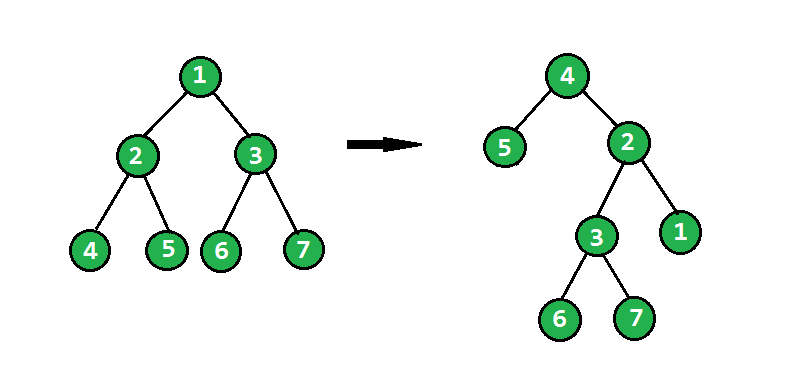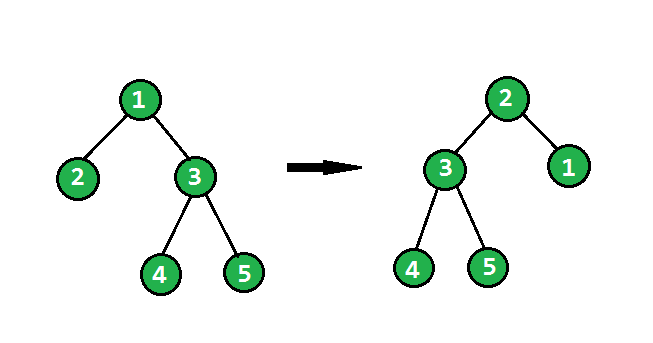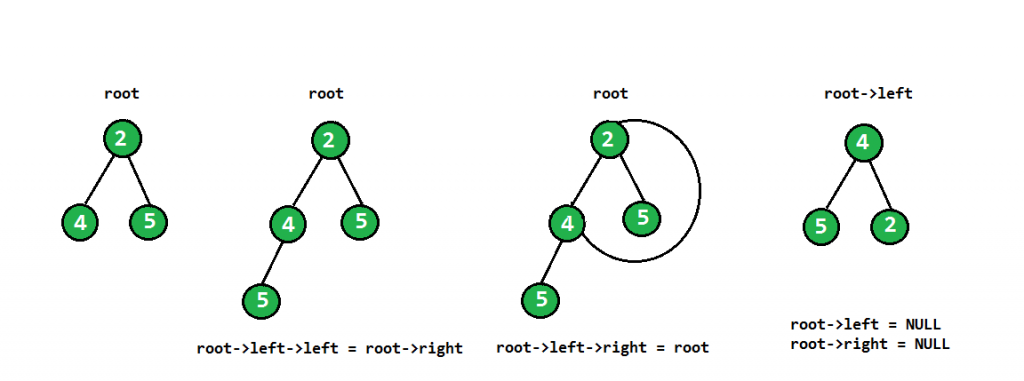Related Articles
Flip Binary Tree
• Difficulty Level : Hard
• Last Updated : 18 Nov, 2020

Given a binary tree, the task is to flip the binary tree towards the right direction that is clockwise. See the below examples to see the transformation.
In the flip operation, the leftmost node becomes the root of the flipped tree and its parent becomes its right child and the right sibling becomes its left child and the same should be done for all left most nodes recursively.Below is the main rotation code of a subtree

```    root->left->left = root->right;
root->left->right = root;
root->left = NULL;
root->right = NULL;
```

The above code can be understood by the following diagram –as we are storing root->left in the flipped root, flipped subtree gets stored in each recursive call.

## C++

 `/*  C/C++ program to flip a binary tree */``#include ``using` `namespace` `std;` `/* A binary tree node structure */``struct` `Node``{``    ``int` `data;``    ``Node *left, *right;``};` `/* Utility function to create a new Binary``   ``Tree Node */``struct` `Node* newNode(``int` `data)``{``    ``struct` `Node *temp = ``new` `struct` `Node;``    ``temp->data = data;``    ``temp->left = temp->right = NULL;``    ``return` `temp;``}` `// method to flip the binary tree``Node* flipBinaryTree(Node* root)``{``    ``// Base cases``    ``if` `(root == NULL)``        ``return` `root;``    ``if` `(root->left == NULL && root->right == NULL)``        ``return` `root;` `    ``//  recursively call the same method``    ``Node* flippedRoot = flipBinaryTree(root->left);` `    ``//  rearranging main root Node after returning``    ``// from recursive call``    ``root->left->left = root->right;``    ``root->left->right = root;``    ``root->left = root->right = NULL;` `    ``return` `flippedRoot;``}` `// Iterative method to do level order traversal``// line by line``void` `printLevelOrder(Node *root)``{``    ``// Base Case``    ``if` `(root == NULL)  ``return``;` `    ``// Create an empty queue for level order traversal``    ``queue q;` `    ``// Enqueue Root and initialize height``    ``q.push(root);` `    ``while` `(1)``    ``{``        ``// nodeCount (queue size) indicates number``        ``// of nodes at current lelvel.``        ``int` `nodeCount = q.size();``        ``if` `(nodeCount == 0)``            ``break``;` `        ``// Dequeue all nodes of current level and``        ``// Enqueue all nodes of next level``        ``while` `(nodeCount > 0)``        ``{``            ``Node *node = q.front();``            ``cout << node->data << ``" "``;``            ``q.pop();``            ``if` `(node->left != NULL)``                ``q.push(node->left);``            ``if` `(node->right != NULL)``                ``q.push(node->right);``            ``nodeCount--;``        ``}``        ``cout << endl;``    ``}``}` `//  Driver code``int` `main()``{``    ``Node* root = newNode(1);``    ``root->left = newNode(2);``    ``root->right = newNode(3);``    ``root->right->left = newNode(4);``    ``root->right->right = newNode(5);` `    ``cout << ``"Level order traversal of given tree\n"``;``    ``printLevelOrder(root);` `    ``root = flipBinaryTree(root);` `    ``cout << ``"\nLevel order traversal of the flipped"``            ``" tree\n"``;``    ``printLevelOrder(root);``    ``return` `0;``}`

## Java

 `/*  Java program to flip a binary tree */``import` `java.util.Queue;``import` `java.util.LinkedList;``public` `class` `FlipTree {` `    ``// method to flip the binary tree``    ``public` `static` `Node flipBinaryTree(Node root)``    ``{``        ``if` `(root == ``null``)``            ``return` `root;``        ``if` `(root.left == ``null` `&& root.right ==``null``)``            ``return` `root;` `        ``//  recursively call the same method``        ``Node flippedRoot=flipBinaryTree(root.left);` `        ``//  rearranging main root Node after returning``        ``// from recursive call``        ``root.left.left=root.right;``        ``root.left.right=root;``        ``root.left=root.right=``null``;``        ``return` `flippedRoot;``    ``}` `    ``// Iterative method to do level order traversal``    ``// line by line``    ``public` `static` `void` `printLevelOrder(Node root)``    ``{``        ``// Base Case``        ``if``(root==``null``)``            ``return` `;``        ` `        ``// Create an empty queue for level order traversal``        ``Queue q=``new` `LinkedList<>();``        ``// Enqueue Root and initialize height``        ``q.add(root);``        ``while``(``true``)``        ``{``            ``// nodeCount (queue size) indicates number``            ``// of nodes at current lelvel.``            ``int` `nodeCount = q.size();``            ``if` `(nodeCount == ``0``)``                ``break``;``            ` `            ``// Dequeue all nodes of current level and``            ``// Enqueue all nodes of next level``            ``while` `(nodeCount > ``0``)``            ``{``                ``Node node = q.remove();``                ``System.out.print(node.data+``" "``);``                ``if` `(node.left != ``null``)``                    ``q.add(node.left);``                ``if` `(node.right != ``null``)``                    ``q.add(node.right);``                ``nodeCount--;``            ``}``            ``System.out.println();``        ``}``    ``}` `    ``public` `static` `void` `main(String args[]) {``        ``Node root=``new` `Node(``1``);``        ``root.left=``new` `Node(``2``);``        ``root.right=``new` `Node(``1``);``        ``root.right.left = ``new` `Node(``4``);``        ``root.right.right = ``new` `Node(``5``);``        ``System.out.println(``"Level order traversal of given tree"``);``        ``printLevelOrder(root);``  ` `        ``root = flipBinaryTree(root);``        ``System.out.println(``"Level order traversal of flipped tree"``);``        ``printLevelOrder(root);``    ``}``}` `/* A binary tree node structure */``class` `Node``{``    ``int` `data;``    ``Node left, right;``    ``Node(``int` `data)``    ``{``        ``this``.data=data;``    ``}``};` `//This code is contributed by Gaurav Tiwari`

## Python3

 `# Python3 program to flip``# a binary tree` `# A binary tree node``class` `Node:``    ` `    ``# Constructor to create``    ``# a new node``    ``def` `__init__(``self``, data):``      ` `        ``self``.data ``=` `data``        ``self``.right ``=` `None``        ``self``.left ``=` `None` `def` `flipBinaryTree(root):``    ` `    ``# Base Cases``    ``if` `root ``is` `None``:``        ``return` `root``    ` `    ``if` `(root.left ``is` `None` `and``        ``root.right ``is` `None``):``        ``return` `root` `    ``# Recursively call the``    ``# same method``    ``flippedRoot ``=` `flipBinaryTree(root.left)` `    ``# Rearranging main root Node``    ``# after returning from``    ``# recursive call``    ``root.left.left ``=` `root.right``    ``root.left.right ``=` `root``    ``root.left ``=` `root.right ``=` `None` `    ``return` `flippedRoot` `# Iterative method to do the level``# order traversal line by line``def` `printLevelOrder(root):``    ` `    ``# Base Case``    ``if` `root ``is` `None``:``        ``return``    ` `    ``# Create an empty queue for``    ``# level order traversal``    ``from` `Queue ``import` `Queue``    ``q ``=` `Queue()``    ` `    ``# Enqueue root and initialize``    ``# height``    ``q.put(root)``    ` `    ``while``(``True``):` `        ``# nodeCount (queue size) indicates``        ``# number of nodes at current level``        ``nodeCount ``=` `q.qsize()``        ``if` `nodeCount ``=``=` `0``:``            ``break` `        ``# Dequeue all nodes of current``        ``# level and Enqueue all nodes``        ``# of next level  ``        ``while` `nodeCount > ``0``:``            ``node ``=` `q.get()``            ``print` `node.data,``            ``if` `node.left ``is` `not` `None``:``                ``q.put(node.left)``            ``if` `node.right ``is` `not` `None``:``                ``q.put(node.right)``            ``nodeCount ``-``=` `1` `        ``print``        ` `# Driver code``root ``=` `Node(``1``)``root.left ``=` `Node(``2``)``root.right ``=` `Node(``3``)``root.right.left ``=` `Node(``4``)``root.right.right ``=` `Node(``5``)` `print` `"Level order traversal of given tree"``printLevelOrder(root)` `root ``=` `flipBinaryTree(root)` `print` `"\nLevel order traversal of the flipped tree"``printLevelOrder(root)` `# This code is contributed by Nikhil Kumar Singh(nickzuck_007)`

## C#

 `// C# program to flip a binary tree``using` `System;``using` `System.Collections.Generic;` `public` `class` `FlipTree``{` `    ``// method to flip the binary tree``    ``public` `static` `Node flipBinaryTree(Node root)``    ``{``        ``if` `(root == ``null``)``            ``return` `root;``        ``if` `(root.left == ``null` `&& root.right ==``null``)``            ``return` `root;` `        ``// recursively call the same method``        ``Node flippedRoot = flipBinaryTree(root.left);` `        ``// rearranging main root Node after returning``        ``// from recursive call``        ``root.left.left = root.right;``        ``root.left.right = root;``        ``root.left = root.right = ``null``;``        ``return` `flippedRoot;``    ``}` `    ``// Iterative method to do level order traversal``    ``// line by line``    ``public` `static` `void` `printLevelOrder(Node root)``    ``{``        ``// Base Case``        ``if``(root == ``null``)``            ``return` `;``        ` `        ``// Create an empty queue for level order traversal``        ``Queue q = ``new` `Queue();``        ` `        ``// Enqueue Root and initialize height``        ``q.Enqueue(root);``        ``while``(``true``)``        ``{``            ``// nodeCount (queue size) indicates number``            ``// of nodes at current lelvel.``            ``int` `nodeCount = q.Count;``            ``if` `(nodeCount == 0)``                ``break``;``            ` `            ``// Dequeue all nodes of current level and``            ``// Enqueue all nodes of next level``            ``while` `(nodeCount > 0)``            ``{``                ``Node node = q.Dequeue();``                ``Console.Write(node.data+``" "``);``                ``if` `(node.left != ``null``)``                    ``q.Enqueue(node.left);``                ``if` `(node.right != ``null``)``                    ``q.Enqueue(node.right);``                ``nodeCount--;``            ``}``            ``Console.WriteLine();``        ``}``    ``}` `    ``// Driver code``    ``public` `static` `void` `Main(String []args)``    ``{``        ``Node root = ``new` `Node(1);``        ``root.left = ``new` `Node(2);``        ``root.right = ``new` `Node(1);``        ``root.right.left = ``new` `Node(4);``        ``root.right.right = ``new` `Node(5);``        ``Console.WriteLine(``"Level order traversal of given tree"``);``        ``printLevelOrder(root);``    ` `        ``root = flipBinaryTree(root);``        ``Console.WriteLine(``"Level order traversal of flipped tree"``);``        ``printLevelOrder(root);``    ``}``}` `/* A binary tree node structure */``public` `class` `Node``{``    ``public` `int` `data;``    ``public` `Node left, right;``    ``public` `Node(``int` `data)``    ``{``        ``this``.data = data;``    ``}``};` `// This code is contributed by Princi Singh`

Output:

```Level order traversal of given tree
1
2 3
4 5

Level order traversal of the flipped tree
2
3 1
4 5

```

Iterative Approach
This approach is contributed by Pal13
The iterative solution follows the same approach as the recursive one, the only thing we need to pay attention to is to save the node information that will be overwritten.

## C++

 `//  C/C++ program to flip a binary tree``#include ``using` `namespace` `std;` `// A binary tree node structure``struct` `Node``{``    ``int` `data;``    ``Node *left, *right;``};` `// Utility function to create a new Binary``// Tree Node``struct` `Node* newNode(``int` `data)``{``    ``struct` `Node *temp = ``new` `struct` `Node;``    ``temp->data = data;``    ``temp->left = temp->right = NULL;``    ``return` `temp;``}` `// method to flip the binary tree``Node* flipBinaryTree(Node* root)``{``    ``// Initialization of pointers``    ``Node *curr = root;``    ``Node *next = NULL;``    ``Node *temp = NULL;``    ``Node *prev = NULL;``    ` `    ``// Iterate through all left nodes``    ``while``(curr)``    ``{``        ``next = curr->left;``        ` `        ``// Swapping nodes now, need temp to keep the previous right child``        ` `        ``// Making prev's right as curr's left child``        ``curr->left = temp;        ``        ` `        ``// Storing curr's right child``        ``temp = curr->right;        ``        ` `        ``// Making prev as curr's right child``        ``curr->right = prev;        ``        ` `        ``prev = curr;``        ``curr = next;``    ``}``    ``return` `prev;``}` `// Iterative method to do level order traversal``// line by line``void` `printLevelOrder(Node *root)``{``    ``// Base Case``    ``if` `(root == NULL) ``return``;` `    ``// Create an empty queue for level order traversal``    ``queue q;` `    ``// Enqueue Root and initialize height``    ``q.push(root);` `    ``while` `(1)``    ``{``        ``// nodeCount (queue size) indicates number``        ``// of nodes at current lelvel.``        ``int` `nodeCount = q.size();``        ``if` `(nodeCount == 0)``            ``break``;` `        ``// Dequeue all nodes of current level and``        ``// Enqueue all nodes of next level``        ``while` `(nodeCount > 0)``        ``{``            ``Node *node = q.front();``            ``cout << node->data << ``" "``;``            ``q.pop();``            ` `            ``if` `(node->left != NULL)``                ``q.push(node->left);``            ` `            ``if` `(node->right != NULL)``                ``q.push(node->right);``            ``nodeCount--;``        ``}``        ``cout << endl;``    ``}``}` `// Driver code``int` `main()``{``    ` `    ``Node* root = newNode(1);``    ``root->left = newNode(2);``    ``root->right = newNode(3);``    ``root->right->left = newNode(4);``    ``root->right->right = newNode(5);` `    ``cout << ``"Level order traversal of given tree\n"``;``    ``printLevelOrder(root);` `    ``root = flipBinaryTree(root);` `    ``cout << ``"\nLevel order traversal of the flipped"``            ``" tree\n"``;``    ``printLevelOrder(root);``    ``return` `0;``}` `// This article is contributed by Pal13`

## Java

 `// Java program to flip a binary tree``import` `java.util.*;``class` `GFG``{``    ` `// A binary tree node``static` `class` `Node``{``    ``int` `data;``    ``Node left, right;``};` `// Utility function to create``// a new Binary Tree Node` `static` `Node newNode(``int` `data)``{``    ``Node temp = ``new` `Node();``    ``temp.data = data;``    ``temp.left = temp.right = ``null``;``    ``return` `temp;``}` `// method to flip the binary tree``static` `Node flipBinaryTree(Node root)``{``    ``// Initialization of pointers``    ``Node curr = root;``    ``Node next = ``null``;``    ``Node temp = ``null``;``    ``Node prev = ``null``;``    ` `    ``// Iterate through all left nodes``    ``while``(curr != ``null``)``    ``{``        ``next = curr.left;``        ` `        ``// Swapping nodes now, need``        ``// temp to keep the previous``        ``// right child``        ` `        ``// Making prev's right``        ``// as curr's left child``        ``curr.left = temp;        ``        ` `        ``// Storing curr's right child``        ``temp = curr.right;        ``        ` `        ``// Making prev as curr's``        ``// right child``        ``curr.right = prev;        ``        ` `        ``prev = curr;``        ``curr = next;``    ``}``    ``return` `prev;``}` `// Iterative method to do``// level order traversal``// line by line``static` `void` `printLevelOrder(Node root)``{``    ``// Base Case``    ``if` `(root == ``null``) ``return``;` `    ``// Create an empty queue for``    ``// level order traversal``    ``Queue q = ``new` `LinkedList();` `    ``// Enqueue Root and``    ``// initialize height``    ``q.add(root);` `    ``while` `(``true``)``    ``{``        ``// nodeCount (queue size)``        ``// indicates number of nodes``        ``// at current lelvel.``        ``int` `nodeCount = q.size();``        ``if` `(nodeCount == ``0``)``            ``break``;` `        ``// Dequeue all nodes of current``        ``// level and Enqueue all nodes``        ``// of next level``        ``while` `(nodeCount > ``0``)``        ``{``            ``Node node = q.peek();``            ``System.out.print(node.data + ``" "``);``            ``q.remove();``            ` `            ``if` `(node.left != ``null``)``                ``q.add(node.left);``            ` `            ``if` `(node.right != ``null``)``                ``q.add(node.right);``            ``nodeCount--;``        ``}``        ``System.out.println();``    ``}``}` `// Driver code``public` `static` `void` `main(String args[])``{``    ``Node root = newNode(``1``);``    ``root.left = newNode(``2``);``    ``root.right = newNode(``3``);``    ``root.right.left = newNode(``4``);``    ``root.right.right = newNode(``5``);` `    ``System.out.print(``"Level order traversal "` `+``                            ``"of given tree\n"``);``    ``printLevelOrder(root);` `    ``root = flipBinaryTree(root);` `    ``System.out.print(``"\nLevel order traversal "` `+``                        ``"of the flipped tree\n"``);``    ``printLevelOrder(root);``}``}` `// This code is contributed``// by Arnab Kundu`

## Python3

 `# Python3 program to flip``# a binary tree``from` `collections ``import` `deque` `# A binary tree node structure``class` `Node:``  ` `    ``def` `__init__(``self``, key):``      ` `        ``self``.data ``=` `key``        ``self``.left ``=` `None``        ``self``.right ``=` `None` `# method to flip the``# binary tree``def` `flipBinaryTree(root):``  ` `    ``# Initialization of``    ``# pointers``    ``curr ``=` `root``    ``next` `=` `None``    ``temp ``=` `None``    ``prev ``=` `None` `    ``# Iterate through all``    ``# left nodes``    ``while``(curr):``        ``next` `=` `curr.left` `        ``# Swapping nodes now, need temp``        ``# to keep the previous right child` `        ``# Making prev's right as curr's``        ``# left child``        ``curr.left ``=` `temp` `        ``# Storing curr's right child``        ``temp ``=` `curr.right` `        ``# Making prev as curr's right``        ``# child``        ``curr.right ``=` `prev` `        ``prev ``=` `curr``        ``curr ``=` `next``    ``return` `prev` `# Iterative method to do level``# order traversal line by line``def` `printLevelOrder(root):``  ` `    ``# Base Case``    ``if` `(root ``=``=` `None``):``        ``return` `    ``# Create an empty queue for``    ``# level order traversal``    ``q ``=` `deque()` `    ``# Enqueue Root and initialize``    ``# height``    ``q.append(root)` `    ``while` `(``1``):``        ``# nodeCount (queue size) indicates``        ``# number of nodes at current level.``        ``nodeCount ``=` `len``(q)``        ``if` `(nodeCount ``=``=` `0``):``            ``break` `        ``# Dequeue all nodes of current``        ``# level and Enqueue all nodes``        ``# of next level``        ``while` `(nodeCount > ``0``):``            ``node ``=` `q.popleft()``            ``print``(node.data, end ``=` `" "``)` `            ``if` `(node.left !``=` `None``):``                ``q.append(node.left)` `            ``if` `(node.right !``=` `None``):``                ``q.append(node.right)``            ``nodeCount ``-``=` `1` `        ``print``()` `# Driver code``if` `__name__ ``=``=` `'__main__'``:``  ` `    ``root ``=` `Node(``1``)``    ``root.left ``=` `Node(``2``)``    ``root.right ``=` `Node(``3``)``    ``root.right.left ``=` `Node(``4``)``    ``root.right.right ``=` `Node(``5``)` `    ``print``(``"Level order traversal of given tree"``)``    ``printLevelOrder(root)` `    ``root ``=` `flipBinaryTree(root)` `    ``print``(``"\nLevel order traversal of the flipped"``          ``" tree"``)``    ``printLevelOrder(root)` `# This code is contributed by Mohit Kumar 29`

## C#

 `// C# program to flip a binary tree``using` `System;``using` `System.Collections.Generic;` `class` `GFG``{` `// A binary tree node``public` `class` `Node``{``    ``public` `int` `data;``    ``public` `Node left, right;``}` `// Utility function to create``// a new Binary Tree Node``public` `static` `Node newNode(``int` `data)``{``    ``Node temp = ``new` `Node();``    ``temp.data = data;``    ``temp.left = temp.right = ``null``;``    ``return` `temp;``}` `// method to flip the binary tree``public` `static` `Node flipBinaryTree(Node root)``{``    ``// Initialization of pointers``    ``Node curr = root;``    ``Node next = ``null``;``    ``Node temp = ``null``;``    ``Node prev = ``null``;` `    ``// Iterate through all left nodes``    ``while` `(curr != ``null``)``    ``{``        ``next = curr.left;` `        ``// Swapping nodes now, need``        ``// temp to keep the previous``        ``// right child` `        ``// Making prev's right``        ``// as curr's left child``        ``curr.left = temp;` `        ``// Storing curr's right child``        ``temp = curr.right;` `        ``// Making prev as curr's``        ``// right child``        ``curr.right = prev;` `        ``prev = curr;``        ``curr = next;``    ``}``    ``return` `prev;``}` `// Iterative method to do level``// order traversal line by line``public` `static` `void` `printLevelOrder(Node root)``{``    ``// Base Case``    ``if` `(root == ``null``)``    ``{``        ``return``;``    ``}` `    ``// Create an empty queue for``    ``// level order traversal``    ``LinkedList q = ``new` `LinkedList();` `    ``// Enqueue Root and``    ``// initialize height``    ``q.AddLast(root);` `    ``while` `(``true``)``    ``{``        ``// nodeCount (queue size)``        ``// indicates number of nodes``        ``// at current lelvel.``        ``int` `nodeCount = q.Count;``        ``if` `(nodeCount == 0)``        ``{``            ``break``;``        ``}` `        ``// Dequeue all nodes of current``        ``// level and Enqueue all nodes``        ``// of next level``        ``while` `(nodeCount > 0)``        ``{``            ``Node node = q.First.Value;``            ``Console.Write(node.data + ``" "``);``            ``q.RemoveFirst();` `            ``if` `(node.left != ``null``)``            ``{``                ``q.AddLast(node.left);``            ``}` `            ``if` `(node.right != ``null``)``            ``{``                ``q.AddLast(node.right);``            ``}``            ``nodeCount--;``        ``}``        ``Console.WriteLine();``    ``}``}` `// Driver code``public` `static` `void` `Main(``string``[] args)``{``    ``Node root = newNode(1);``    ``root.left = newNode(2);``    ``root.right = newNode(3);``    ``root.right.left = newNode(4);``    ``root.right.right = newNode(5);` `    ``Console.Write(``"Level order traversal "` `+``                         ``"of given tree\n"``);``    ``printLevelOrder(root);` `    ``root = flipBinaryTree(root);` `    ``Console.Write(``"\nLevel order traversal "` `+``                     ``"of the flipped tree\n"``);``    ``printLevelOrder(root);``}``}` `// This code is contributed by Shrikant13`

Output:

```Level order traversal of given tree
1
2 3
4 5

Level order traversal of the flipped tree
2
3 1
4 5

```

Complexity Analysis:
Time complexity: O(n) as in the worst case, depth of binary tree will be n.
Auxiliary Space: O(1).

https://www.youtube.com/watch?v=S8GZFCbst2g

This article is contributed by Utkarsh Trivedi. If you like GeeksforGeeks and would like to contribute, you can also write an article using contribute.geeksforgeeks.org or mail your article to contribute@geeksforgeeks.org. See your article appearing on the GeeksforGeeks main page and help other Geeks.
Please write comments if you find anything incorrect, or you want to share more information about the topic discussed above.

Attention reader! Don’t stop learning now. Get hold of all the important DSA concepts with the DSA Self Paced Course at a student-friendly price and become industry ready.

My Personal Notes arrow_drop_up